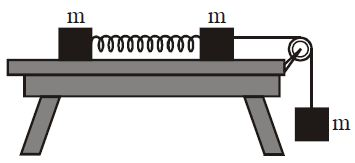# Blocks on table!

At a smooth horizontal table are two identical cubes of mass $m$, connected by a spring of rigidity $k$. The length of spring in the un-stretched state is $l_0$. The right cube is linked to the load mass $m$ at the end. At some time the system is released and the system moves without initial velocity. Find the maximum distance (in cm) between blocks during the motion of the system.

[$l_0 = 1 cm$, $m = 3 kg$, $k = 1000 N/m$]×

Problem Loading...

Note Loading...

Set Loading...